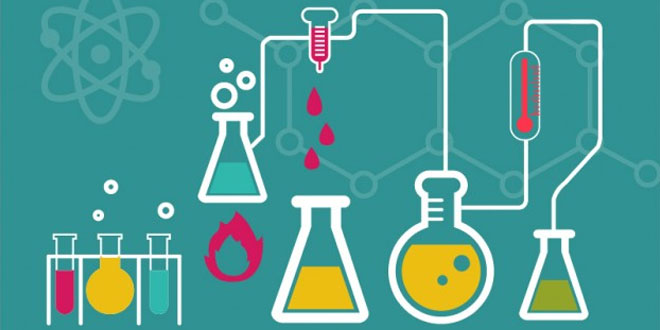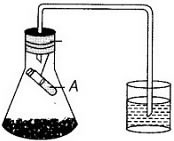Tuesday , May 26 2020# 10 Class Science Periodic Test II (2018-19)

## 10 Class Science Periodic Test II (2018-19)

School Name: Venkateshwar Global School, Sector 13, Rohini, Delhi 110085 India
Time: 3 hour
M.M. 80 marks
Date: 10/09/2018
Class: X

## General Instructions:

1. The question paper comprise two sections, A and B. You are to attempt both the sections.
2. All questions are compulsory.
3. All questions of Section-A and B are to be attempted separately.
4. There is an internal choice in two questions of three marks each and one question of five marks.
5. Question numbers 1 & 2 in Section – A are 1 mark question. They are to be answered in one word or in one sentence.
6. Question numbers 3 to 5 in Section – A are 2 marks questions. These are to be answered in 30 words each.
7. Question numbers 6 to 15 in Section – A are 3 marks questions. These are to be answered in about 50 words each.
8. Question numbers 16 to 21 in Section – A are 5 marks questions. These are to be answered in 70 words each.
9. Question numbers 22 to 27 in Section – B are  based on practical skills. Each question is a two marks question. These are to be answered in brief.

## Section A:

#### 4. Define relative refractive index of a medium B with respect to medium A in terms of

1. speed of light in these media and
2. their refractive indices nd nB.

#### 7. (i) A potted plant is growing in a transparent glass jar. In this plant X and Y are two growing  parts having a lot of materialistic tissue. It is observed that the part X of this plant exhibits geotropism but phototropism. On the other hand, part Y of this plant exhibits negative geotropism but positive phototropism. (2+1)

1. Name the part X of plant.
2. Name the part Y of plant.
3. Which part of the plant, X or Y, will exhibit positive hydro-tropism?
4. Which part of the plant, X or Y, can have tendrils on It?

#### (ii) Use the suitable words and complete the statements given below.

1. Any change in the organism’s environment that causes it to react is a _______.
2. The reaction of the organism to the change in its environment is the _______ .

#### 8. (i) Draw the diagram of cross – section of a leaf and label the following in it:

1. Chloroplast
2. Guard cell
3. Lower epidermis
4. Upper epidermis

#### 11. (i) The pH of soil A is 7.5 while that of soil B 4.5. Which of the two soils A or B should be treated with chalk powder to adjust its pH and why? (ii) State reason for the following: (1+2)

1. For dilution of acid, acid is added into water and not water into acid.
2. Ammonia is a base but does not contain hydroxyl group.

#### 15. If in the figure R1=10Ω, R2=40Ω, R3=30Ω, R4=20Ω, R5=60Ω, and a 12 V battery is connected to the arrangement, calculate

1. the total resistance of the circuit,
2. the total current flowing in the circuit, and
3. heat produced in 2 minutes.#### 17. What is atmospheric refraction? Use this phenomenon to explain the following  natural events.

1. Twinkling of stars.
2. Advanced sunrise and delayed  sunset.

#### 18. A metal E is stored under kerosene. When a small piece of it is left open in the air, it catches fire.  When the product formed is dissolved in water, it turns litmus to blue.

1. Name the metal E.
2. Write the chemical equation for the reaction when it is exposed to air and when the product is dissolved in water.
3. Explain the process with equation involved, by which the metal E is obtained from its molten chloride.   (1+2+2)

#### 19. (i) A metal nitrate A on heating gives yellowish brown colored metal oxide along with brown gas B and a colorless gas C. Aqueous solution of a on reaction with potassium iodide forms a yellow precipitate of compound D.

1. Identify  A, B, C and D.
2. Write balanced chemical equations for both the reactions. Also identify the types of reactions.

## Section – B (Practical Based Questions)

#### 24. The diagram given below is the experimental set-up to show that carbon dioxide is given out during respiration. In this set-up what does test tube marked (A) contain? What is its role in the experiment?## NCERT 8th Class CBSE Science: Friction

Friction: 8th Class NCERT CBSE Science Chapter 12 Question: What is friction? Answer: Friction is a …

### One comment

1.Nice awesome very help full!